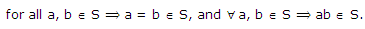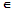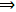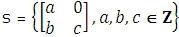# What is a subring in algebra

Publish On: 2019-03-17

Total Post: 571

# Mjay Jollay

Total Post: 0

## ANS: What is a subring in algebra

Stable for the composition in the Ring R

Let (R, +, . >) be a ring and let S be a non-empty subset of R, i.e. S ⊂ R. Then S is stable for the addition and multiplication composition in R ifInduced composition

Let a non-empty subset S of the ring (R, +, . >) be stable for the addition and multiplication compositions defined in R. Then we say that the compositions ‘+’, ‘.‘ in R have induced compositions in S. These compositions in S are called induced compositions.

Subring

Let (R, +, .) be a ring, then a non-empty subset S ⊂ R is called a subring of R, if S is stable for the compositions in R and S itself is a ring with respect to these induced compositions. In other words, a non-empty subset S of a ring (R, +, .) if

(i) ∀ a, bSa + bS andabS.

(ii) (S, +, .) forms a ring.

Examples of subrings

1. The ring of even integers is a subring of the ring of integers (Z, +, .).

2. The ring of rational numbers is a subring of the ring of real numbers (R, +, .).

3.The ring of Gaussian integers is a subring of the ring of complex numbers (C, +, .).

4. The set of all real valued differentiable functions on [0, 1] is a subring of the ring of all real-valued continuous functions.

5. The set mZ of multiples of integers by a non-zero number m, m≠0 is a subring of (Z, +, .).

6. The ring of integers is a subring of the ring of rational numbers (Q, +, .).

7. The setis a subring of all 2 × 2 matrices over Z.